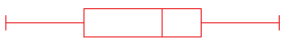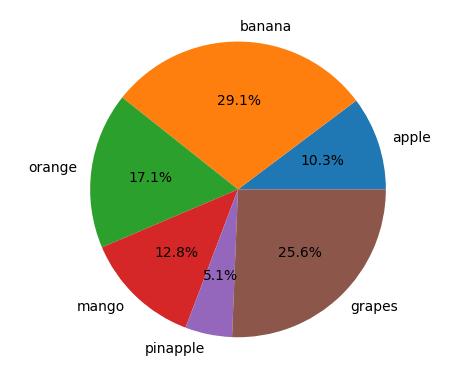# Data Visualization MCQ Class 12 IP | Plotting with PyplotThis post contains Multiple Choice based Questions with answers for Data Visualization MCQ Class 12 IP. These objective questions with answers are not only helpful for those students preparing for CBSE IP Class 12 Python Plotting With Pyplot MCQ but also for all those students who are practicing and learning Python Programming specially Data Handling and Visualization using Python Matplotlib Plotting with pyplot .

You will get different categories of Questions for all the concept of Python Matplotlib Plotting with pyplot objective questions-

Assertion and Reason MCQs
Case Study Based MCQs
Find The Output Based MCQs
Concept Based MCQs

#### Data Visualization MCQ Class 12 IP SET-3 (Q51-Q75)

1. The value of _________ chart is calculated in terms of percentage.

a. Histogram
b. Boxplot
c. Bar
d. Pie

d. Pie

1. By default, Plot() function plots a

a. Bar chart
b. Pie chart
c. Line chart
d. Horizontal bar chart

c. Line chart

1. Which of the following option describes a column chart?

a. Comparison in different categories of data
b. Relationship between two sets of numeric data
c. Contribution of individual values to a total value
d. Data trend over a period of time

a. Comparison in different categories of data

1. Which of the following is not a method to create a chart using pyplot?

a. pie()
b. barh()
c. plot()
d. histg()

d. histg()

1. To represent  proportionate contribution of individual data we can use ______ chart

a. Line
b. Bar
c. Pie
d. Scatter

c. Pie

1. Symbol which represent single data value(point) in a chart is called

a. Ticks
b. Marker
c. Title
d. Data labels

b. Marker

1. Which of the following is best suitable chart to show data correlation?

a. Bar
b. Histogram
c. Pie
d. Scatter

d. Scatter

1. Raju want to create scatter chart for the given data

X=[1,2,3,4]
Y= [12,23,16,28]

Help him to write correct code (important libraries are imported)

a. Plt.plot(x,y,’o’)
b. Plt.scatter(x,y)
c. Df=pd.DataFrame({‘c1’:x,’c2’:y})
Df.plot(kind=scatter,x=’c1’,y=’c2’)
d. a and b both
e. All of the above

e. All of the above

1. Pyplot is a

a. Module
b. Library
c. Function
d. Collection

a. Module

1. The default type of histogram is

a. Line
b. Line with bar
c. Bar
d. bar with dots

c. Bar

1. Which of the following argument cannot used with hist()?

a. Bin
b. Width
c. Histtype
d. Cumulative

a. Bin

1. By default hist() uses bin value of _____

a. 5
b. 10
c. 15
d. 20

b. 10

1. Which of the following website provides data freely to download and to do data analysis for educational purpose?

a. data.gov.in
b. digitalindia.gov.in
c. data.mygov.in
d. csc.gov.in

a. data.gov.in

1. _____ chart display only one data series at a time.

a. Line
b. Bar
c. Pie
d. Scatter

c. Pie

1. Which of the following data summary is not included in box plot?

a. Median
b. Quartile
c. Count
d. Maximum

c. Count

1.  represents aa. Frequency polygon
b. Histogram
c. Box plot
d. Scatter plot

c. Box Plot

1. Box plot can be created by writing command

a. Plt.box()
b. Plt.boxplot()
c. Plt.plot(kind=’box’)
d. All of the above

b. Plt.boxplot()

1. which of the following is correct statement to save a chart using savefig()

a. plt.savefig(“graph.png”)
b. plt.savefig(“graph.jpg”)
c. plt.savefig(“graph.pdf”)
d. plt.savefig(“graph.ppt”)

d. plt.savefig(“graph.ppt”)

1. if the extention of file name is not given in savefig(), than file is saved with _____ extention

a. png
b. jpg
c. pdf
d. eps

a. png

1. From the following dataset
63,65,67,69,69,70,71,71,73,74,74,75,77,77,83,85,87
Create a horizontal boxplot (important libraries are included)

a. L=[63,65,67,69,69,70,71,71,73,74,74,75,77,77,83,85,87]
plt.boxplot(L, horiz = True)
plt.show()

b. L=[63,65,67,69,69,70,71,71,73,74,74,75,77,77,83,85,87]
plt.boxplot(L, orientation = False)
plt.show()

c. L=[63,65,67,69,69,70,71,71,73,74,74,75,77,77,83,85,87]
plt.boxplot(L, vert = False)
plt.show()

d. All are correct

```c. L=[63,65,67,69,69,70,71,71,73,74,74,75,77,77,83,85,87]
plt.boxplot(L, vert = False)
plt.show()```

1. Select the correct statement:

(i) We can specify width argument containing sequence to specify different width of the bars in bar chart
(ii) If x and y limits for a plot are not set , pyplot automatically sets the limits as per values of dataset

a. Both are true
b. Both are false
c. (i) true (ii) false
d. (i) false (ii) true

a. Both are true

1. Write appropriate code for given chart (important libraries are already included)a. a=[‘apple’,’banana’,’orange’,’mango’,’pinapple’,’grapes’]
b=[120,340,200,150,60,300]
plt.pie(a,b, autopct = ‘%1.1f%%’)
plt.show()

b. a=[‘apple’,’banana’,’orange’,’mango’,’pinapple’,’grapes’]
b=[120,340,200,150,60,300]
plt.pie(b,a, autopct = ‘%1.1f%%’)
plt.show()

c. a=[‘apple’,’banana’,’orange’,’mango’,’pinapple’,’grapes’]
b=[120,340,200,150,60,300]
plt.pie(b,labels = a, autopct = ‘%1.1f%%’)
plt.show()

d. a=[‘apple’,’banana’,’orange’,’mango’,’pinapple’,’grapes’]
b=[120,340,200,150,60,300]
plt.pie(a,labels = b, autopct = ‘%1.1f%%’)
plt.show()

```c. a=['apple','banana','orange','mango','pinapple','grapes']
b=[120,340,200,150,60,300]
plt.pie(b,labels = a, autopct = '%1.1f%%')
plt.show()```

1. predict the output of given code

import pandas as pd
import matplotlib.pyplot as plt
x=[2,4,6,8]
y=[23,10,45,20]
plt.plot(y,x,color=’m’,linestyle = ‘dashed’,marker  = ‘+’, markersize = 10, markeredgecolor = ‘b’)
plt.show()

a.b.c. No Output
d. Error

a.

1. Which of the following is used to set the limit of x-axis of the plot?

a. Limit()
b. Limits()
c. Xlimit()
d. Xlimits()

d. Xlimits()

1. Argument used in pie() to create wedges out from the pie chart is

a. Wedge
b. Explode
c. Slice
d. fillet